1. Math Worksheets>
2. Measurement>
3. Time>
4. Time Conversion

# Time Conversion: Days, Hours, Minutes and Seconds

The worksheets in this page contain time conversion between days and hours; hours and minutes; minutes and seconds. You also have mixed review under each type. Adding and subtracting times worksheets included for additional practice.

Converting Hours and Minutes: Level 1

Compute how many minutes can make the hours and vice versa.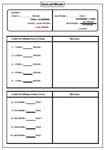Hours to Minutes:

Minutes to Hours:

Mixed Review:

Converting Hours and Minutes: Level 2

Each worksheet has 16 problems converting hours plus minutes into minutes and vice versa.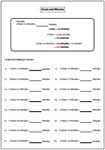Converting into Minutes:

Converting into Hours:

Mixed Review:

Converting Minutes and Seconds: Level 1

1 minute = 60 seconds. Use this fact to solve each worksheet.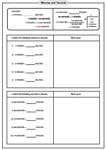Minutes to Seconds:

Seconds to Minutes:

Mixed Review:

Converting Minutes and Seconds: Level 2

Converting time worksheets between minutes and seconds.Converting to Seconds:

Converting to Minutes:

Mixed Review:

Converting Days and Hours: Level 1

Each worksheets 10 practice problems converting between days and hours. Note that 12 hours equivalent to half a day.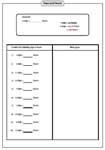Days to hours:

Hours to days:

Mixed Review:

Converting Days and Hours: Level 2

Click the preview below to see how level 2 is different from level 1.Converting to hours:

Converting to days:

Mixed Review:

Add the time duration. Level 1 has sum of the minutes less than 60, but level 2 contains the sum of the minutes more than 60.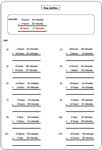Level 1:

Level 2:

Grab 'em All

Subtracting Time

Subtract the time duration. Level 1 and level 2 are based on 'no borrowing' and 'borrowing' respectively.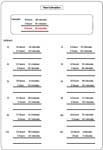Level 1: No Borrowing

Level 2: Borrowing

Grab 'em All

The entire collection of time conversion worksheets can be downloaded in a jiffy!

Related Worksheets